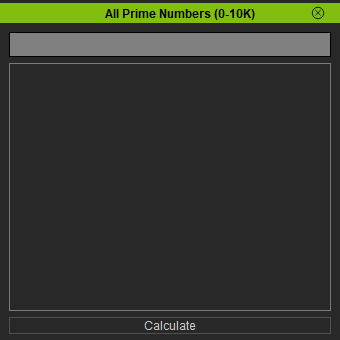# Difference between revisions of "IC Python API:Progress Bar"

## Contents

Main article: RL Python Samples.

This lesson will go over the creation of a progress bar as one of the fundamental building blocks of a functional user interface.

## Required Modules

```import RLPy
from PySide2 import QtWidgets
from PySide2.shiboken2 import wrapInstance
```

## Prime Number Checker

For our CPU intensive operation, we'll be checking for prime numbers from 1 to 10,000. A prime number is natural number greater than 1 that cannot be formed by multiplying two smaller natural numbers. The opposite of this is a composite number. For example, 5 is prime because the only ways of writing it as a product, 1 × 5 or 5 × 1, involve 5 itself. However, 6 is composite because it is the product of two numbers (2 × 3) that are both smaller than 6.

```def check_prime_number(num):
if num > 1:    # Prime numbers are greater than 1
for i in range(2, num):     # Check for factors
if (num % i) == 0:
return False
else:
return True
else:     # If input number is less than or equal to 1, it is not prime
return False
```

## Building the UI

```window = RLPy.RUi.CreateRDockWidget()
window.SetWindowTitle("All Prime Numbers (0-10K)")

dock = wrapInstance(int(window.GetWindow()), QtWidgets.QDockWidget)
dock.setFixedSize(350, 350)
dock.setStyleSheet(
""" QProgressBar{ font: bold; color: black; border: 1px solid black; background-color: grey;}
QProgressBar::chunk { width: 1px; background-color: #13c1ec}""")

widget = QtWidgets.QWidget()
dock.setWidget(widget)

layout = QtWidgets.QVBoxLayout()
widget.setLayout(layout)

progress_bar = QtWidgets.QProgressBar()

button = QtWidgets.QPushButton("Calculate")
button.clicked.connect(run)

for widget in [progress_bar, text_edit, button]:

window.Show()
```

Notice the Calculate button runs a function to start the entire prime number search process which is covered below.

## Running the Script

Keep in mind that progress bars may not always run smoothly. Particularly when the operations are very taxing on the system, one may experience fits and starts from time to time.```def run():

progress_bar.setRange(1, 10000)
button.setHidden(True)
found = 0

for i in range(1, 10001):
if check_prime_number(i):
text_edit.insertPlainText("{:,}".format(i) + "\t")
found += 1
progress_bar.setValue(i)
progress_bar.setFormat(f"Calculating: {round(i/100)}%")
bottom = text_edit.verticalScrollBar().maximum()
text_edit.verticalScrollBar().setValue(bottom)

progress_bar.setFormat(f"{ '{:,}'.format(found) } prime numbers found.")
```

## Everything Put Together

```import RLPy
from PySide2 import QtWidgets
from PySide2.shiboken2 import wrapInstance

def check_prime_number(num):
if num > 1:    # Prime numbers are greater than 1
for i in range(2, num):     # Check for factors
if (num % i) == 0:
return False
else:
return True
else:     # If input number is less than or equal to 1, it is not prime
return False

def run():

progress_bar.setRange(1, 10000)
button.setHidden(True)
found = 0

for i in range(1, 10001):
if check_prime_number(i):
text_edit.insertPlainText("{:,}".format(i) + "\t")
found += 1
progress_bar.setValue(i)
progress_bar.setFormat(f"Calculating: {round(i/100)}%")
bottom = text_edit.verticalScrollBar().maximum()
text_edit.verticalScrollBar().setValue(bottom)

progress_bar.setFormat(f"{ '{:,}'.format(found) } prime numbers found.")

window = RLPy.RUi.CreateRDockWidget()
window.SetWindowTitle("All Prime Numbers (0-10K)")

dock = wrapInstance(int(window.GetWindow()), QtWidgets.QDockWidget)
dock.setFixedSize(350, 350)
dock.setStyleSheet(
""" QProgressBar{ font: bold; color: black; border: 1px solid black; background-color: grey;}
QProgressBar::chunk { width: 1px; background-color: #13c1ec}""")

widget = QtWidgets.QWidget()
dock.setWidget(widget)

layout = QtWidgets.QVBoxLayout()
widget.setLayout(layout)

progress_bar = QtWidgets.QProgressBar()